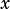(x + 2)^2 + (y – 3)^2 = 40
y = -2x + 4

Which could be the x-coordinate of a solution to the system of equations above?

A) √7

B) √35 / 2

C) (6 + 2√34) /5

D) (4 + 2√191) /5

Let’s substitute. Use the second equation to make the first equation all-values.Well, not exactly a walk in the park, but it all comes together nicely! A is the answer.